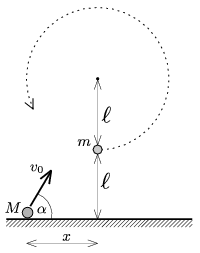Mathematical and Physical Journal
for High Schools
Issued by the MATFUND Foundation
 Already signed up? New to KöMaL?

#Problem P. 4384. (November 2011)

P. 4384. A small ball of mass m=0.1 kg is attached to a=1 m-long thread and is hung to a horizontal peg. The small ball is at rest and another small ball of mass M=0.2 kg, is projected from the ground and collides with it, such that the collision is totally elastic and head on, and after the collision the ball at the end of the thread completes a whole circle around the peg. The distance between the peg and the ground is 2.

a) What is the least distance of x shown in the figure?

b) What is the initial velocity of the projected ball? (Speed and direction.)(5 pont)

Deadline expired on December 12, 2011.

Sorry, the solution is available only in Hungarian. Google translation

Megoldás. $\displaystyle a)$ $\displaystyle x\ge 2{,}37$ m.

$\displaystyle b)$ $\displaystyle \alpha=40{,}2^\circ$ és $\displaystyle v_{0}=6{,}87~$m/s.

### Statistics:

 98 students sent a solution. 5 points: 53 students. 4 points: 17 students. 3 points: 25 students. 2 points: 2 students. 1 point: 1 student.

Problems in Physics of KöMaL, November 2011